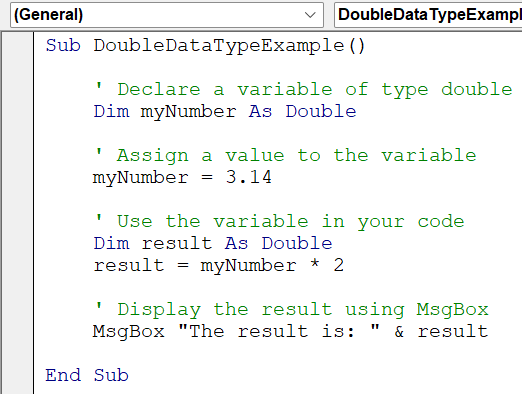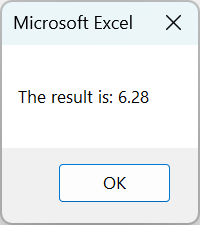# VBA CDBL FunctionArticle byExcelMojo Team## What is Excel VBA CDBL Function?

The Excel VBA CDBL Function converts a given expression or value into a double data type. It stands for “Convert to Double.” This function is beneficial when dealing with calculations or operations requiring precise decimal accuracy, as the double data type can handle floating-point numbers with high precision.

Let’s look at an example that demonstrates the conversion of an integer value to a double using the VBA CDBL function.

The code begins by declaring two variables. The “intValue” variable is assigned a value of 10. Then, the VBA CDBL function converts the integer value to a double, and the result is stored in the “dblValue” variable.

A message box displays the converted double value, showing “10.0.”

Note: The output of the message box for the above code will display “10” instead of “10.0”. This is because when a double value is displayed using MsgBox, it is automatically formatted to its most concise representation without trailing zeros.

###### Key Takeaways
• The VBA CDBL function converts an expression or value to the double data type.
• Examples of using the VBA CDBL function include converting strings to doubles, handling type mismatch errors, and converting integers to doubles.
• Some important points to remember about the VBA CDBL function are the possibility of type mismatch errors, limitations on precision, and the influence of regional settings.

### How to Use a Double Data Type in VBA Coding?

To use a double data type in VBA coding, follow these steps:

1. Create a subroutine “DoubleDataTypeExample()” and declare a variable of type double by using the “Dim” statement. For example:

Sub DoubleDataTypeExample()
Dim myNumber As Double

2. Assign a value to the variable. You can do this using the equal sign (=). For example:

myNumber = 3.14

3. Use the variable in your code for calculations or other operations. For example:

Dim result As Double
result = myNumber * 2

4. Display the result using the message box.

MsgBox “The result is: ” & result
End Sub

5. When you run this code, it will display a message box with the calculated result, of “myNumber” (3.14) by 2.### Examples

Here, we have a few examples which depict how to use VBA CBDL in different scenarios.

#### Example #1

In this example, we will look at a VBA subroutine that converts a string value to a double using the VBA CDBL Function and displays the resulting double value using a message box.

• Step 1:
• Open the VBE.
• Press the Alt + F11 keys in Excel.
• Click on the Developer tab in the ribbon.
• Click on the Visual Basic button.
• Step 2: In the VBE, click the Insert menu and select Module to create a new module.
• Step 3: Start a new subroutine. Begin a new subroutine named “ConvertStringToDouble().”
• Step 4: In this step, two variables are declared: “strNumber” as a String type and “dblNumber” as a Double type. These variables will be used to store the string and converted double values, respectively.
• Step 5: The string variable “strNumber” is assigned “3.14.” This value is a string representation of a numeric value.
• Step 6: The VBA CDBL function converts the string value stored in “strNumber” to a double. The VBA CDBL function takes the string as input and returns the equivalent double value, which is then assigned to the “dblNumber” variable.
• Step 7: The MsgBox function displays a message box containing the value stored in “dblNumber”. In this case, it will display the converted double value of 3.14.
• Step 8: Now, save the module and close the VBE. Press Alt + F8 to open the Macro window, select “ConvertStringToDouble” and run it.
• Step 9: When you run this code, a message box displays the value 3.14 as a double data type.

Here is the full code:

Sub ConvertStringToDouble()
‘ Declare variables
Dim strNumber As String
Dim dblNumber As Double
‘ Assign a value to the string variable
strNumber = “3.14”
‘ Convert the string to a double using CDbl function
dblNumber = CDbl(strNumber)
‘ Display the double value using MsgBox
MsgBox dblNumber
End Sub

#### Example #2

In this example, we will look at a VBA subroutine that handles a type mismatch error when attempting to convert a variant value to a double using the VBA CDBL function.

• Step 1: Start a new subroutine named “HandleTypeMismatchError()” in the new module.
• Step 2: Two variables are declared: “value” as a Variant type and “dblNumber” as a Double type. The “value” variable will store a value that might cause a type mismatch error, and “dblNumber” will store the converted double value.
• Step 3: Now, assign a value to the variant variable. The variant variable “value” is assigned the value “Hello.” It is a non-numeric string, intentionally chosen to demonstrate a potential type mismatch error.
• Step 4: We attempt to convert the variant to a double using the VBA CDBL function. The “On Error Resume Next” statement handles potential errors during the conversion. It allows the program to continue execution without halting at the error line.

The VBA CDBL function converts the value stored in the “value” variable to a double. Since “Hello” cannot be converted to a double, it will result in a type mismatch error.

However, the error is ignored due to the “On Error Resume Next” statement and the program continues to execute.

• Step 5: Check for a type mismatch error:
• After attempting the conversion, the code checks if there is a type mismatch error by examining the “Err.Number” property.
• An error occurs during the conversion if the “Err.Number” is non-zero.
• In that case, a message box is displayed with the text “Type mismatch error!” to notify the user about the error.
• The “Err.Clear” statement is used to clear the error object, and the “On Error GoTo 0” statement resets the error handling behavior to the default mode.
• Step 6: Now, save the module and close the VBE. Press Alt + F8 to open the Macro window, select “HandleTypeMismatchError” and run it.
• Step 7: When you run this code, it will trigger a Type Mismatch Error in VBA when attempting to convert the string “Hello” to a double. A message box will display the “Type mismatch error!” message.

Here is the full code:

Sub HandleTypeMismatchError()
‘ Declare variables
Dim value As Variant
Dim dblNumber As Double
‘ Assign a value to the variant variable
value = “Hello”
‘ Attempt to convert the variant to a double using CDbl function
On Error Resume Next
dblNumber = CDbl(value)
‘ Check for a type mismatch error
If Err.Number <> 0 Then
MsgBox “Type mismatch error!”
Err.Clear
On Error GoTo 0
End If
End Sub

#### Example #3

In this example, we will look at a VBA subroutine that converts an integer value to a double using the VBA CDBL function and displays the resulting double value using a message box.

• Step 1: Start a new subroutine:

Begin a new subroutine named “ConvertIntegerToDouble().”

• Step 2: Two variables are declared: “intValue” as an Integer type and “dblValue” as a Double type. The “intValue” variable will store an integer value, and “dblValue” will store the converted double value.
• Step 3: The integer variable “intValue” is assigned the value 10. This is a numeric value that can be easily converted to a double without any loss of precision.
• Step 4: The VBA CDBL function converts the value stored in the “intValue” variable to a double. The VBA CDBL function takes the integer value as input and returns the equivalent double value, which is then assigned to the “dblValue” variable.
• Step 5: The “MsgBox” function is used to display a message box containing the value stored in “dblValue.” In this case, it will display the converted double value of 10.0.
• Step 6: Now, save the module and close the VBE. Now press Alt + F8 to open the Macro window, select “ConvertIntegerToDouble” and Run.
• Step 7: When you run this code, a message box displaying the value 10.0 as a double data type will appear.

Here is the full code:

Sub ConvertIntegerToDouble()
‘ Declare variables
Dim intValue As Integer
Dim dblValue As Double
‘ Assign a value to the integer variable
intValue = 10
‘ Convert the integer to a double using CDbl function
dblValue = CDbl(intValue)
‘ Display the double value using MsgBox
MsgBox dblValue ‘ Displays 10.0 as a double
End Sub

### Important Things to Note

• It’s crucial to ensure that the input value to the VBA CDBL function is appropriate for conversion to a double to avoid errors.
• The VBA CDBL Function may encounter a type mismatch error if the input expression cannot be converted to a double. It is essential to handle such errors using proper error-handling techniques.
• The precision of double values is limited and may only be exact for some decimal values. Using the Decimal data type is recommended when precise decimal accuracy is required.
• The CDBL Function follows the default number format specified in Excel. Ensuring that the regional settings are configured correctly is essential to avoid any unexpected conversions.
• Troubleshooting the VBA CDBL function involves validating input data, reviewing error handling, checking regional settings, and testing with different data to identify and resolve any issues.

1. What is a type mismatch with CDBL VBA in Excel?

A type mismatch error occurs when the input expression provided to the CDBL function cannot be converted to a double data type. This error commonly happens when attempting to convert a non-numeric value or a string that cannot be interpreted as a numeric value to a double.

2. What is the difference between CDbl and Val in Excel VBA?

The main difference between CDBL and Val in Excel VBA lies in the way they handle non-numeric input. The VBA CDBL function will raise a type mismatch error if the input expression cannot be converted to a double. On the other hand, the Val function attempts to extract any leading numeric characters from a string and converts it to a double. If the input string does not start with a valid numeric value, Val returns 0.

3. Why is the Excel VBA CDBL function not working?

The Excel VBA CDBL function may not work correctly if:
The input expression or value cannot be converted to a double data type.
The regional settings in Excel are not configured correctly, resulting in unexpected conversions.
There is an issue with the input data validation or error handling in the code. It is crucial to validate the input data and handle potential errors appropriately to ensure the CDBL function works as intended.

This has been a guide to VBA CDBL Function. Here we learn to convert value to double data type & handle type mismatch error using CDbl function, with examples. You can learn more from the following articles –